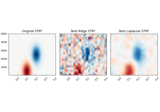# mne.decoding.TimeDelayingRidge#

class mne.decoding.TimeDelayingRidge(tmin, tmax, sfreq, alpha=0.0, reg_type='ridge', fit_intercept=True, n_jobs=None, edge_correction=True)[source]#

Ridge regression of data with time delays.

Parameters:
tmin

The starting lag, in seconds (or samples if `sfreq` == 1). Negative values correspond to times in the past.

tmax

The ending lag, in seconds (or samples if `sfreq` == 1). Positive values correspond to times in the future. Must be >= tmin.

sfreq`float`

The sampling frequency used to convert times into samples.

alpha`float`

The ridge (or laplacian) regularization factor.

reg_type

Can be `"ridge"` (default) or `"laplacian"`. Can also be a 2-element list specifying how to regularize in time and across adjacent features.

fit_interceptbool

If True (default), the sample mean is removed before fitting.

n_jobs

The number of jobs to use. Can be an int (default 1) or `'cuda'`.

New in v0.18.

edge_correctionbool

If True (default), correct the autocorrelation coefficients for non-zero delays for the fact that fewer samples are available. Disabling this speeds up performance at the cost of accuracy depending on the relationship between epoch length and model duration. Only used if `estimator` is float or None.

New in v0.18.

Notes

This class is meant to be used with `mne.decoding.ReceptiveField` by only implicitly doing the time delaying. For reasonable receptive field and input signal sizes, it should be more CPU and memory efficient by using frequency-domain methods (FFTs) to compute the auto- and cross-correlations.

Methods

 `fit`(X, y) Estimate the coefficients of the linear model. `get_params`([deep]) Get parameters for this estimator. Predict the output. `set_params`(**params) Set the parameters of this estimator.
fit(X, y)[source]#

Estimate the coefficients of the linear model.

Parameters:
X`array`, shape (n_samples[, n_epochs], n_features)

The training input samples to estimate the linear coefficients.

y`array`, shape (n_samples[, n_epochs], n_outputs)

The target values.

Returns:
selfinstance of `TimeDelayingRidge`

Returns the modified instance.

get_params(deep=True)[source]#

Get parameters for this estimator.

Parameters:
deepbool, optional

If True, will return the parameters for this estimator and contained subobjects that are estimators.

Returns:
params`dict`

Parameter names mapped to their values.

predict(X)[source]#

Predict the output.

Parameters:
X`array`, shape (n_samples[, n_epochs], n_features)

The data.

Returns:
X`ndarray`

The predicted response.

set_params(**params)[source]#

Set the parameters of this estimator.

The method works on simple estimators as well as on nested objects (such as pipelines). The latter have parameters of the form `<component>__<parameter>` so that it’s possible to update each component of a nested object.

Parameters:
**params`dict`

Parameters.

Returns:
instinstance

The object.

## Examples using `mne.decoding.TimeDelayingRidge`#Spectro-temporal receptive field (STRF) estimation on continuous data

Spectro-temporal receptive field (STRF) estimation on continuous data